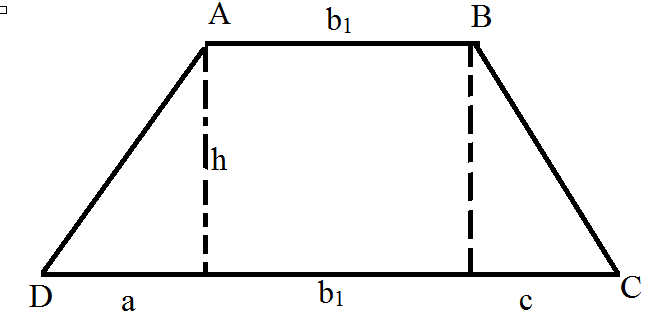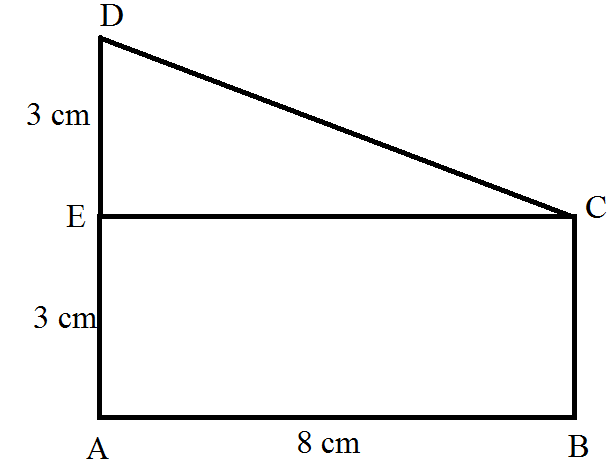QuestionAnswers

# Find the area of trapezium $ABCD$ as given in the figure in which $ADCE$ is a rectangle.Verified
129k+ views
Hint: A trapezium is a quadrilateral which is defined as a shape with four sides and one set of parallels side.
The line segment connecting the midpoints of the non-parallel sides of a trapezoid is called the mid-segment.
If we drew a line segment, between the two non-parallels sides. From the mid-point of both sides, the trapezium will be divided into two unequal parts.Area$= \dfrac{k}{2}(AB + CD)$
We know that
Area of trapezoid $=$area of triangle (1)$+$ area of rectangle $+$area of triangle (2)
That means
$A = \dfrac{{ah}}{2} + bh + \dfrac{{ch}}{2}$
$A = \dfrac{{ah + 2{b_1}h + cb}}{2}$
Simplifying the equation, rearranging the term and factoring result to
$A = \dfrac{h}{2}\left[ {{b_1}\_(a + {b_1}\_c)} \right].........(1)$
We assume the longer base of the trapezium be ${b_2}$ then
${b_2} = a + {b_1} + c...........(2)$
Substituting (2) in equation (1)
$A = \dfrac{h}{2}\left( {{b_1} + {b_2}} \right)$
Therefore, the arc of trapezium with b bases ${b_1} + {b_2}$ and altitude h is
$A = \dfrac{h}{2}\left( {{b_1} + {b_2}} \right)$
Therefore

Given image= where ADCE is a rectangleGiven
$BE = 3cm$ $AB = 3 + 3 = 6cm$
$EA = 3cm$ $CD = 3cm$
$AD = 8cm$ $AD = 8cm$
Area of trapezium $ABCD = \dfrac{1}{2} \times$ parallel sides $\times$height
$= \dfrac{1}{2} \times (AB + CD) \times AD$
$= \dfrac{1}{2} \times (6 + 3) \times 8$ {BY putting the value of AB, CD and Ad in the formula}
$= \dfrac{1}{2} \times 9 \times 8 = 36c{m^2}$
Hence,
The area of the trapezium
$ABCD = 36c{m^2}$

Note: Properties of trapezium
The sum of all the four angles of the trapezium is equal to ${360^0}$
It has two parallels and two non-parallels sides
The diagonals of regular trapezium bisect each other
The length of the mid-segment is equal to half the sum of the parallel’s bases, in trapezium
Two Paris of adjacent angles of trapezium formed between the parallel’s sides and one of the non-parallels side, add up to 180 degrees.